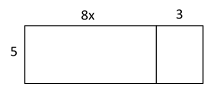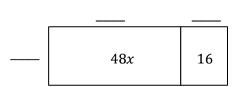# Numerical and Algebraic Expressions

## Objective

Expand and factor expressions using the distributive property and the greatest common factor.

## Common Core Standards

### Core Standards

?

• 7.EE.A.1 — Apply properties of operations as strategies to add, subtract, factor, and expand linear expressions with rational coefficients.

?

• 6.EE.A.3

• 7.NS.A.2

## Criteria for Success

?

1. Use the distributive property to expand an expression by distributing a term through a sum or difference in parentheses.
2. Model the distributive property using rectangular arrays and partial areas.
3. Factor out a greatest common factor from a sum or difference and write a new expression as a product.
4. Understand that ${a(b+c)=ab+ac}$.

## Tips for Teachers

?

In Lessons 3 and 4, students expand and factor expressions with rational numbers. Students have prior experience with using the distributive property to generate equivalent expressions from 6th grade (6.EE.3). In Lesson 3, students see negative numbers inside a parentheses group written as subtraction, however, they will not distribute with a negative number outside a parentheses group until Lesson 4.

### Remote Learning Guidance

If you need to adapt or shorten this lesson for remote learning, we suggest prioritizing Anchor Problems 1 and 2 (benefit from worked examples) and Anchor Problem 3 (can be done independently). Find more guidance on adapting our math curriculum for remote learning here.

#### Fishtank Plus

• Problem Set
• Student Handout Editor
• Vocabulary Package

## Anchor Problems

?

### Problem 1

Write two equivalent expressions to represent the total area of the rectangle shown. Justify that your two expressions are equivalent.Expand the expression below by using a rectangular array.

${\frac{1}{3}(9x-12y-18)}$

#### References

EngageNY Mathematics Grade 7 Mathematics > Module 3 > Topic A > Lesson 3Example 3

Grade 7 Mathematics > Module 3 > Topic A > Lesson 3 of the New York State Common Core Mathematics Curriculum from EngageNY and Great Minds. © 2015 Great Minds. Licensed by EngageNY of the New York State Education Department under the CC BY-NC-SA 3.0 US license. Accessed Dec. 2, 2016, 5:15 p.m..

Modified by Fishtank Learning, Inc.

### Problem 2

What could be the missing dimensions of the rectangular array? Which set of dimensions uses the greatest common factor of the two terms?Factor the expression below by using a rectangular array. Use the greatest common factor as the representation of the width.

${24d-8e+6}$

#### References

EngageNY Mathematics Grade 7 Mathematics > Module 3 > Topic A > Lesson 4Exercise 2

Grade 7 Mathematics > Module 3 > Topic A > Lesson 4 of the New York State Common Core Mathematics Curriculum from EngageNY and Great Minds. © 2015 Great Minds. Licensed by EngageNY of the New York State Education Department under the CC BY-NC-SA 3.0 US license. Accessed Dec. 2, 2016, 5:15 p.m..

Modified by Fishtank Learning, Inc.

### Problem 3

The table below includes expressions that are written in expanded form and in factored form. Complete the table. Use rectangular arrays as needed.

 Factored Form Expanded Form $3(x-14y)$ $2x+3x$ $\frac{3}{4}(8a+16b)$ $24m+8n-12p$ $\frac{1}{2}\left(\frac{1}{3}x-8y+\frac{2}{3}z\right)$ $5xy+15y$ $2x(-3+y)$

## Problem Set

?The following resources include problems and activities aligned to the objective of the lesson that can be used to create your own problem set.

?

If we multiply ${\frac{x}{2}+\frac{3}{4}}$ by $4$, we get ${{2x+3}}$

Is ${{2x+3}}$ an equivalent expression to ${\frac{x}{2}+\frac{3}{4}}$? Explain your answer.